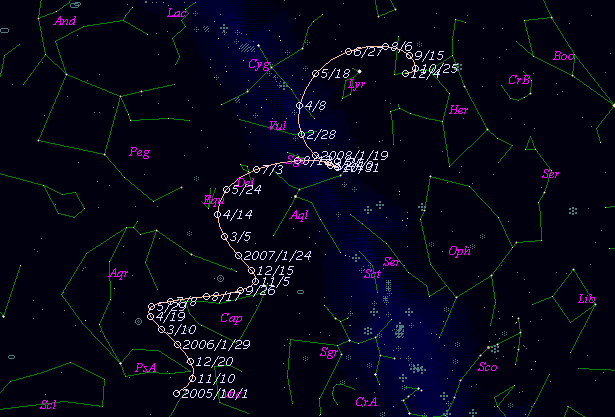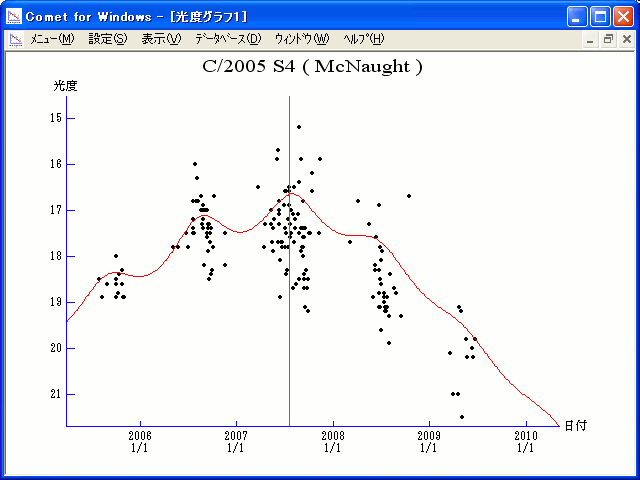# \$B%^%/%N!<%HWB@1(B

C/2005 S4 ( McNaught )###\$B%W%m%U%#!<%k(B

 \$BH/8+F|(B 2005\$BG/(B9\$B7n(B30\$BF|(B \$BH/8+8wEY(B 18.9\$BEy(B \$BH/8+ R. H. McNaught (Siding Spring Survey)

###\$B###\$B50F;MWAG(B

```Epoch 2007 June 29.0 TT = JDT 2454280.5
T 2007 July 18.67707 TT                                 MPC
q   5.8500878            (2000.0)            P               Q
z  +0.0001799      Peri.   31.45691     +0.52975056     -0.56457841
+/-0.0000009      Node   318.29016     -0.82841392     -0.18436035
e   0.9989474      Incl.  107.95860     +0.18191954     +0.80452625
From 520 observations 2005 July 27-2009 July 23, mean residual 0".6.
```

###\$B@1?^(B###\$B8wEYJQ2=(B

```        m1 =  5.5 + 5 log\$B&\$(B + 10.0 log r  [ ,0]  (              \$B!A(B2007\$BG/(B 7\$B7n(B18\$BF|(B)
m1 = -2.2 + 5 log\$B&\$(B + 20   log r  [0, ]  (2007\$BG/(B 7\$B7n(B18\$BF|!A(B              )
```##### \$B50F;MWAG\$O(BM.P.E.C. 2014-E18\$B\$K7G:\\$5\$l\$?\$b\$N\$G\$9!#(B \$B@1?^\$O(B StellaNavigator Ver.2.0 for Windows (\$B%"%9%H%m%"!<%D(B \$BJTCx(B / \$B%"%9%-!<=PHG6I4)(B) \$B\$G:n@.\$7\$?\$b\$N\$G\$9!#(B \$B8wEY%0%i%U\$O(BComet for Windows\$B\$G:n@.\$7\$?\$b\$N\$G\$9!#(B